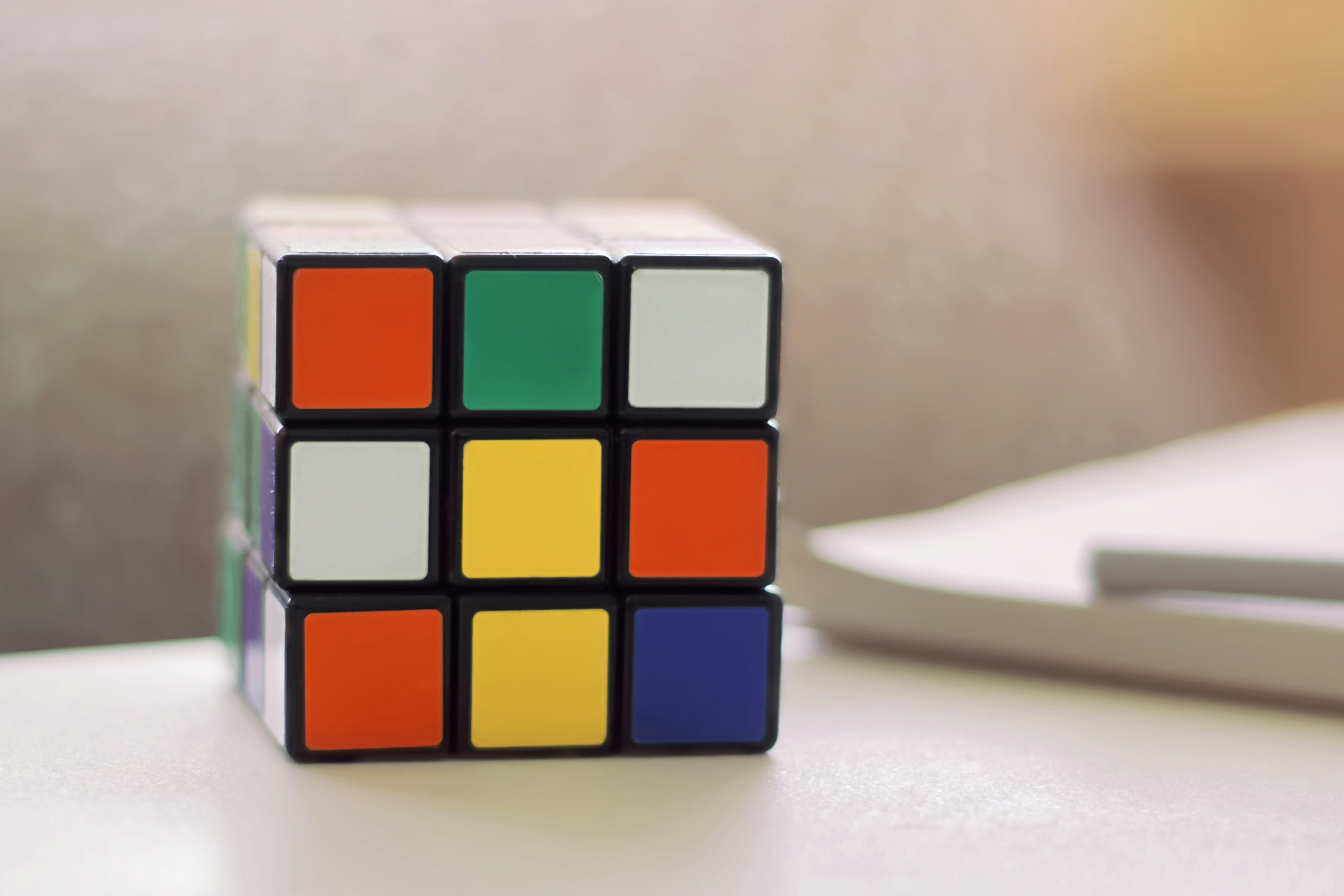Sherlock and Permutations

# Sherlock and PermutationsGiven a string S of N 0's and M 1's, how many unique permutations of this string start with 1?

Help Sherlock by printing the answer modulo (109+7).

Input Format
First line contains T, the number of test cases.
Each test case consists of N and M separated by a space.

Output Format
For each test case, print the answer modulo (109+7).

Constraints
1 ≤ T ≤ 200
1 ≤ N,M ≤ 1000

Sample Input

2
1 1
2 3


Sample Output

1
6


Explanation
Test1: Out of all unique permutations ie. 01 and 10, only second permutation satisfies. Hence, output is 1.
Test2: Out of all unique permutations ie. 00111 01011 01101 01110 10011 10101 10110 11001 11010 11100, only 10011 10101 10110 11001 11010 11100 satisfy. Hence, output is 6.

### Solution in Python

#!/bin/python3

import os
import sys

def facdiv(m,n):
p = 1
for i in range(m+1,n+1):
p*=i
return p

def solve(n, m):
m-=1
nums=[n,m]
nums.sort()
n,m = nums

for _ in range(int(input())):
n,m = list(map(int,input().split()))
print(solve(n,m))